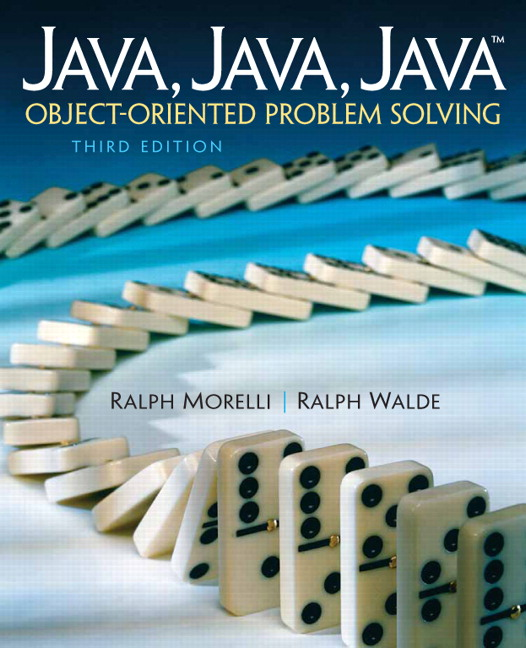# Java, Java, Java: Object-Oriented Problem Solving, 2022E

## Section12.10Chapter Summary

### Subsection12.10.1Technical Terms

 base case recursion parameter computational overhead recursive case head-and-tail algorithm recursive definition iterative method recursive method last-in-first-out (LIFO) self-similarity method call stack tail recursive

### Subsection12.10.2Important Points

• A recursive definition is one that defines the nth case of a concept in terms of the $$(n-1)$$st case plus a limiting condition. It is based on the idea of breaking a problem up into smaller, self-similar problems.
• A recursive method is one that calls itself. It is usually defined in terms of a base case or limiting case, which stops the recursive process, and a recursive case, which breaks the method into a smaller, self-similar copy of itself. A recursion parameter is generally used to control the recursion.
• An iterative algorithm is one that uses some kind of loop as its control structure. Any algorithm that can be done iteratively can also be done recursively, and vice versa.
• Because method calling is relatively costly both in terms of memory used and CPU time involved, a recursive algorithm is generally less efficient than an iterative one that does the same thing.
• In designing recursive algorithms, the base case defines a limit. Each level of recursion should make progress toward the limit, and the algorithm should eventually reach the limit. The limit is usually expressed in terms of the recursion parameter.
• A recursive method is tail recursive if and only if each of its recursive calls is the last action executed by the method.
• A Swing JComboBox component is used to represent a GUI drop-down menu.